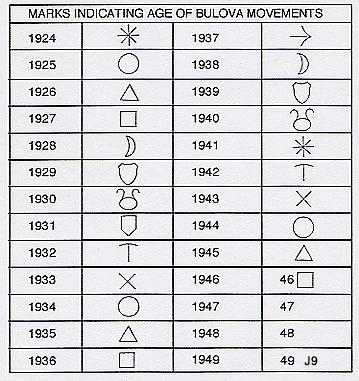BULOVA INFO
Bulova Date Codes
From 1924 until the end of 1940’s Bulova used a series of symbols and letters marked on the movement to indicate the year of manufacture. Beginning in the 1950’s (with some examples from the late 40’s) a series of codes, with a letter/number combination, was marked on the case back to denote the year of manufacture. The letter indicates decade and number indicates year. The last few years of the 1940’s was a transitional period and both systems were in use. Examples of movments stamped with 47, 48 or 49 have been documented as well as watches with the code “A8” and “A9” stamped on case back.Date marks Bulova movements 1924 – 49

Bulova case back date codes
A8 = 1948
A9 = 1949
L1=1951
L2=1952
L3=1953
L4=1954
L5=1955
L6=1956
L7=1957
L8=1958
L9=1959
M0=1960
M1=1961
M2=1962
M3=1963
M4=1964
M5=1965
M6=1966
M7=1967
M8=1968
M9=1969
N0=1970
N1=1971
N2=1972
N3=1973
N4=1974
N5=1975
N6=1976
N7=1977
N8=1978
N9=1979
P0=1980
P1=1981
P2=1982
P3=1983
P4=1984
P5=1985
P6=1986
P7=1987
P8=1988
P9=1989
T0=1990
T1=1991
T2=1992
T3=1993
T4=1994
T5=1995
T6=1996
T7=1997
T8=1998
T9=1999

Bulova Caliber Numbers

a.The number digit signifies the Ligne size of the movement.
b. The next two alpha digits (as below “AE”, “AA”, “CS”) have no real
meaning other than a reference ( like a name).
c. The third and fourth, and sometimes fifth alpha digit would signify the
functional characteristics of the movement.

Letter “C” means “center second hand”
Letter “A” means “automatic”
Letter “B” means “day and date display”
Letter “D” means “date only”

For example:
Movement number “10BSACB”
This would mean –
10 ligne size movement
“BS” family
Center second hand
Automatic wind
DayDate display.

If there are only two letters, then
the watch does not have the characteristics mentioned above.

Categories News & Information